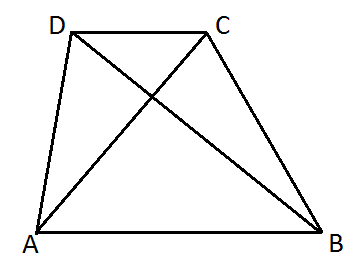# RS Aggarwal Class 8 Math Fifteenth Chapter Quadrilaterals Exercise 15 Solution

## EXERCISE 15

(1) Fill in the blanks:

(i) A quadrilateral has four sides.

(ii) A quadrilateral has four angles.

(iii) A quadrilateral has four vertices, no three of which are collinear.

(iv) A quadrilateral has two diagonals.

(v) A diagonal of a quadrilateral is a line segment that joins two opposite vertices of the quadrilateral.

(vi) The sum of the angles of a quadrilateral is 360o.(i) How many pairs of adjacent sides are there? Name them.

Ans: Four; (AB, BC), (BC, CD), (CD, DA), (DA, AB)

(ii) How many pairs of opposite sides are there? Name them.

Ans: Two; (AB, DC), (AD, BC)

(iii) How many pairs of adjacent angles are there? Name them.

Ans: Four, (∠A, ∠B), (∠B, ∠C), (∠C, ∠D), (∠D, ∠A)

(iv) How many pairs of opposite angles are there? Name them.

Ans: Two; (∠A, ∠C), (∠B, ∠D)

(v) How many diagonals are there? Name them.

Ans: Two; (AC, BD)

(3) Prove that the sum of a quadrilateral is 360o.Solution: Let ABCD be a quadrilateral. Join AC.

Clearly, ∠1 + ∠2 = ∠A ……….(i)

And, ∠3 + ∠4 = ∠C ………..(ii)

We know that the sum of the angles of a triangle is 180o.

∴ From ∆ ABC, we have

∠1 + ∠3 + ∠B = 180o

From ∆ACD, we have

∠1 + ∠3 + ∠D = 180o

Adding the angles on either side, we get:

∠2 + ∠4 + ∠B + ∠1 + ∠3 + ∠D = 360o

⇒ (∠1 + ∠2) + ∠B + (∠3 + ∠4) + ∠D = 360o

⇒ ∠A + ∠B + ∠C + ∠D = 360o (proved)

(4) The three of a quadrilateral are 76o, 54o and 108o. Find the measure of the fourth angle.

Solution: Let the measure of the fourth angle be xo.

∴ 76 + 54 + 108 + x = 360

⇒ x + 238 = 360

⇒ x = 360 – 238

⇒ x = 122

Hence, the measure of the fourth angle is 122o.

(5) The angles of a quadrilateral in the ratio 3 : 5 : 7 : 9. Find the measure of each of these angles.

Solution: Let the angles of the quadrilateral are 3x, 5x, 7x and 9x.

∴ 3x + 5x + 7x + 9x = 360

⇒ 24x = 360

⇒ x = 15

Therefore, measure of each angles are

3 × 15 = 45o

5 × 15 = 75o

7 × 15 = 105o

9 × 15 = 135o.

(6) A quadrilateral has three acute angles, each measuring 75o. Find the measure of the fourth angle.

Solution: Let the measure of the fourth angle be xo.

∴ x + (75 × 3) = 360

⇒ x + 225 = 360

⇒ x = 360 – 225

⇒ x = 135

Therefore, measure of the fourth angle is 135o.

(7) Three angles of a quadrilateral are equal and the measure of the fourth angle is 120o. Find the measure of each of equal angles.

Solution: Let the measure of each equal angle be xo.

∴ x + x + x + 120 = 360

⇒ 3x = 360 – 120

⇒ 3x = 240

⇒ x = 80

Therefore, the measure of each of the equal angle is 80o.

(8) Two angles of a quadrilateral measure 85o and 75o respectively. The other two angles are equal. Find the measure of each of these equal angles.

Solution: Let the measure of each equal angle be xo.

∴ x + x + 85 + 75 = 360

⇒ 2x + 160 = 360

⇒ 2x = 360 – 160

⇒ 2x = 200

⇒ x = 100

Therefore, the measure of each equal angle is 100o.

(9) In the adjacent figure, the bisectors of ∠A and ∠B meet in a point P. If ∠C = 100o and ∠D = 60o, find the measure of ∠APB.Solution: Here,But, ∠BAP + ∠ABP + ∠APB = 180o

⇒ 100o + ∠APB = 180o

⇒ ∠APB = 180o – 100o = 80o

Updated: May 30, 2022 — 2:32 pm